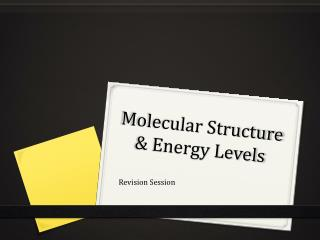DownloadDownload PresentationMolecular Structure & Energy Levels

# Molecular Structure & Energy Levels

Télécharger la présentation## Molecular Structure & Energy Levels

- - - - - - - - - - - - - - - - - - - - - - - - - - - E N D - - - - - - - - - - - - - - - - - - - - - - - - - - -
##### Presentation Transcript

1. Molecular Structure & Energy Levels Revision Session

2. Quantum Mechanics The de Broglie Hypothesis where p is particle momentum (mass x velocity) Heisenberg Uncertainty Relation • N: Normalisation Factor • : Probability • : Integrating across all space

3. Quantum Mechanics What does ψ look like? The wavefunction is finiteeverywhere Does not tend to infinity The wavefunction must be single valued Does not bend back on itself The wavefunction must be continuous Does not ‘kink’ or have sharp angles

4. Quantum Mechanics Eigenvalues : Operator f: Eigenfunction g: Eigenvalue (constant) of the Operator

5. Quantum Mechanics Particle in a 1-D Box

6. Example Example What is the probability of finding the particle in the middle third of the box in the n = 1 state?

7. Example

8. Quantum Mechanics

9. Quantum Mechanics

10. Past Questions

11. Past Questions

12. Quantum Mechanics Electronic Spectra of Polyenes Consider butadiene Take average carbon-carbon bond length as 0.14 nm There are 4 pi electrons, filling n=1 and 2 levels What is the energy of the first transition (n=2  n=3)?

13. Quantum Mechanics Electronic Spectra of Polyenes